Project showcase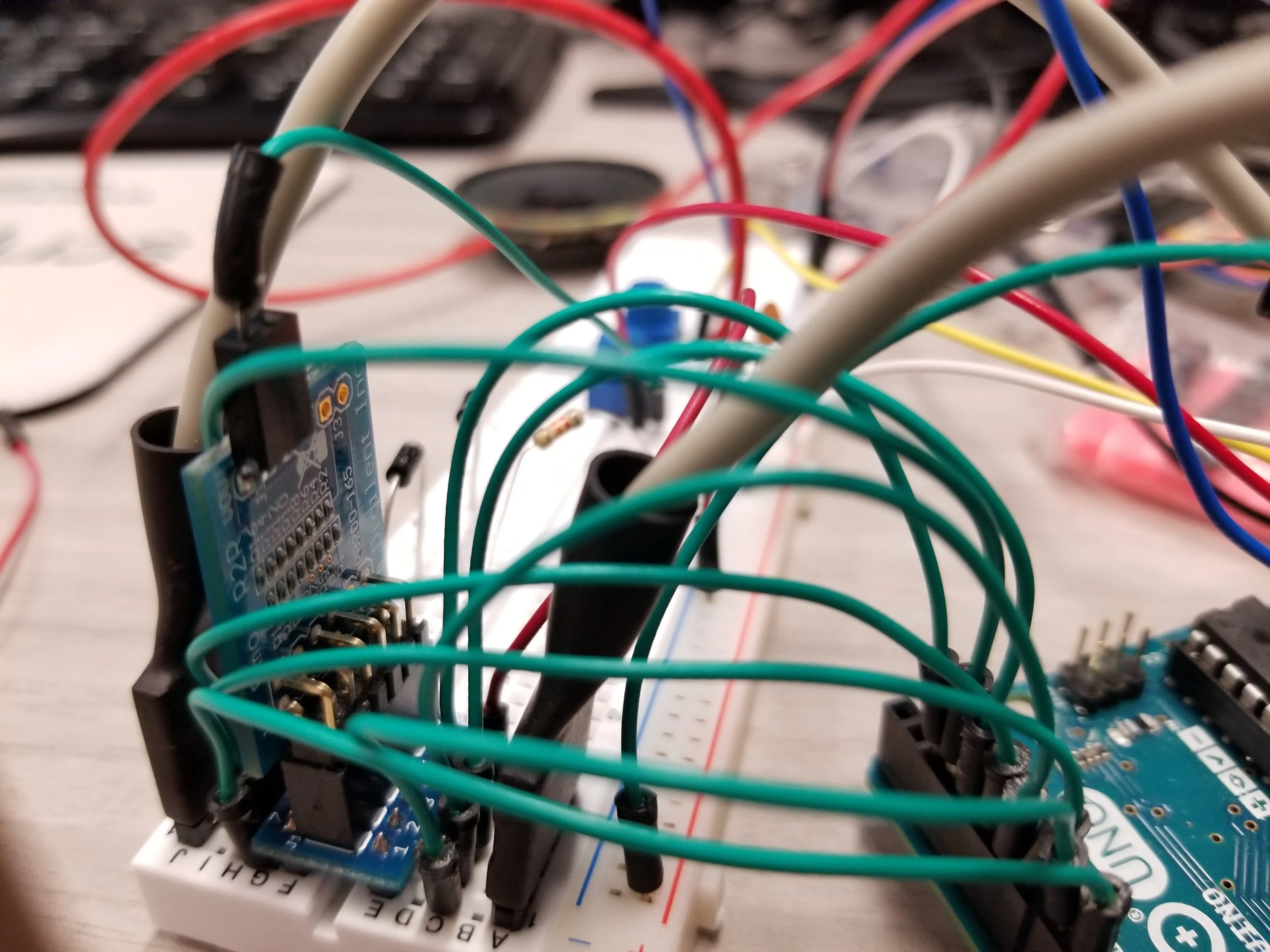Generating Audio with an Arduino and a Resistor Ladder DAC © MIT

I wasn't happy with the way the square waves sound, so I explored using other methods of generating sound waves with Arduino.

• 5,728 views
• 13 respects

Components and supplies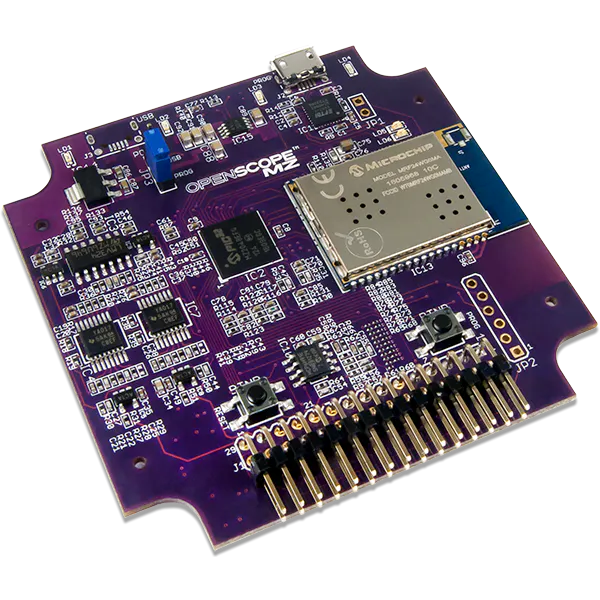Digilent OpenScope MZ
×1Arduino UNO & Genuino UNO
×1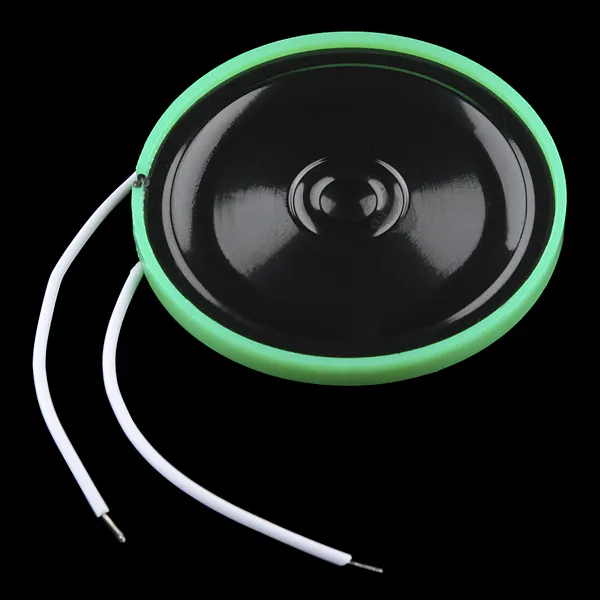Speaker: 0.25W, 8 ohms
×1Capacitor 100 nF
×1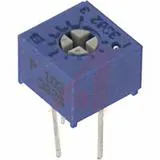Single Turn Potentiometer- 10k ohms
×1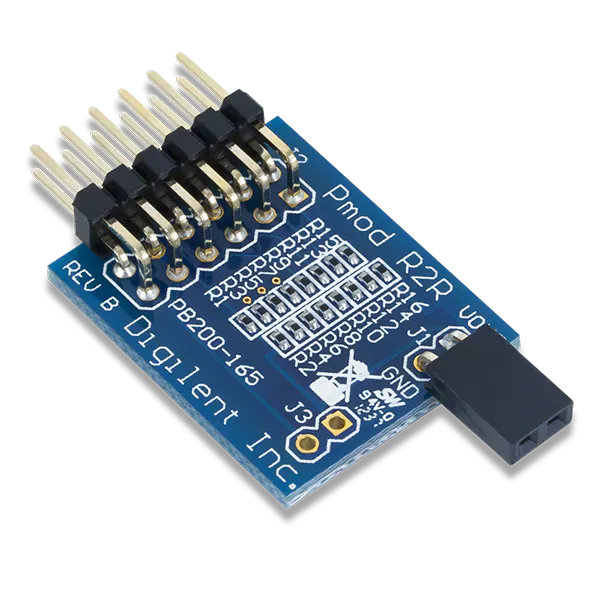Digilent Pmod R2R
×1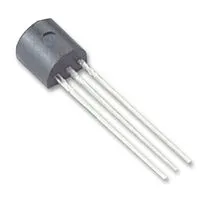General Purpose Transistor NPN
×1

Apps and online services

 Digilent WaveForms Live

Introduction

Arduino has a built-in function for generating tones, and there's a library on GitHub that allows users to expand the number of simultaneous tones that can be played from one to three. However, since the Arduino doesn't come with DACs to generate truly analog signals, both the built-in function and add-on libraries use square waves to generate tones. As my previous article about digital sound synthesis discusses, square waves have a particular sound due to the fact they are composed of odd harmonics. It's not physically possible to create a pure tone composed of only one frequency with a square wave. This makes the sound of a square wave hollow and woody.

Creating an Analog Signal

In order to create an analog signal, I needed a digital-to-analog converter (DAC). Basically, a DAC takes a binary number and converts it to some specific voltage. An 8-bit DAC has 256 (00000000 to 11111111) different voltage levels across the reference voltage range. There are many types of DACs, each using a different method for generating analog outputs. One of the least technical ones, and one I had access to, is implemented in an 8-bit R2R DAC Pmod from Digilent.

R2R DACs use a structure called a resistor ladder to create discrete voltage outputs, as seen in the schematic below.

Each pin numbered D0 to D7 is connected to a different digital pin on the Arduino. Voltage levels are selected by setting the pins to represent a binary number. Since the maximum output will be 255, equaling to 5V in the case of Arduino, any value below that will be the fractional value of 5V. So 2.5V will be represented by 128 (half of 256 levels: 0 to 255) or b1000000. I created an 8-bit R2R DAC in Falstad to help understand how it works. The signals are ordered D0 to D7 from bottom to top.

It's possible to create triangle or sine waves with this DAC, which will have a harmonic composition that sounds more natural than square waves. In the Arduino code, I used two for-loops to set the values of 8 different output pins, and iterated sequentially through the values up to 255 in one loop, and down to 0 in the other. This process creates a triangle wave.

The schematic below shows how to connect the R2R DAC to the Arduino and the speaker. The pin connection pattern is set to reflect the code, so if you change it be sure to change the order in the code as well.

Trouble with Timing

The specific way a note sounds is a result of the fundamental frequencies it's composed of. Therefore, the amount of time it takes to generate one period of a signal will determine the frequency at which it oscillates. Since the process of setting each pin and iterating through for-loops takes several clock cycles of the processor, the maximum frequency that can be generated with the R2R DAC is limited by the number of steps taken in each loop. I found that after optimizing my code, I was only able to synthesize a signal at about 35Hz, which is just above the threshold for hearing. This is directly related to the amount of time it takes the Arduino to cycle through 512 states, setting each of the 8 pins high or low for each state. A lot of time could be saved by reducing the number of states the Arduino cycles through. Playing with the Falstad simulation, I realized that the first four bits of the code were only responsible for varying the bottom 292mV, meaning that I could leave them all high and still have over 4V of signal range. The trade-off is that my signal would be less smooth, as the remaining 4V would only have 16 states (as I would only need to vary the four most-significant bits). Implementing these changes, I was able to generate a signal at 1130Hz, a fairly large improvement over the previous, barely-audible, signal.

Generating Accurate Tones

In order to accurately generate a variety of tones, I calculated the time it takes the Arduino to iterate through a single period (one for-loop up, and one down) without any delays. The value turned out to be 1100 microseconds. So to generate a tone at any given frequency, I'd needed to distribute a delay across all 32 voltage levels contained in a single period of the signal. The formula for each delay (in microseconds) between the levels was the following:

Delay = (1,000,000/[desired Hz] - 1100)/32

I found that this formula was able to create tones that were pretty close to the correct frequencies, although I didn't check every note in the range.

Comparing to the Tones Library

I already had an Arduino sketch that used the Github tones library linked at the beginning of this article. It used two arrays to play a melody: one for the notes (frequencies) and the other for the note duration. I'd programmed the first few stanzas of the melody from Beethoven's Moonlight Sonata's 1st Movement, so I used the same arrays to play the melody using my DAC method and the tone library. Aside from expected differences in volume (the DAC signal's voltage amplitude isn't as high as the pulses from the tones library), the sound had a different quality. The DAC sound wasn't as "digital" sounding, and had a more organic quality to it. However, it seems like some of the notes weren't as accurate, so the melody was more "in tune" when using the tones library. For example, A4, which is considered to be 440Hz, played at 437Hz with the DAC.

I used an OpenScope and WaveForms Live to look at the Fast Fourier Transform (FFT) of the same notes generated by a square wave and a triangular wave. It became clear that the difference in sound quality resulted from differing harmonic compositions. In the images below, the yellow line is the DAC signal, while the blue line represents the square wave.

The DAC signal is almost completely composed of the primary frequency (440Hz), with a nearly non-existent third harmonic. The square wave has a much stronger odd-harmonic composition.

On the technical side, the tones library uses hardware timers to implement a variable-frequency PWM signal. This means that a tone can be played while the Arduino is doing other things, and the frequency will not be affected by adding more commands. In my code, every additional line came at the cost of a reduced bandwidth, and therefore fewer notes being available. After the calculations necessary to play a melody from an array, the highest frequency my code could generate is around 920Hz, or just shy of A5-sharp, whereas the tones library can go as high as 65.535kHZ, or more than 3 times the maximum frequency humans can hear.

In Conclusion

I think for most uses, the tones library is an excellent choice. Not only does it allow for the Arduino to play a tone continuously, but it can play up to three tones simultaneously through a single speaker. This is much more than my code can hope to achieve. The only advantage my process has over this is that the tones are more pleasant to listen to, because they resemble natural sounds more than square waves. However, this was still an interesting project because I was able to see how each line of code would affect the speed of the waves, which was a code-optimization challenge.

by 3 developers

• 3,207 views
• 7 respects

Detecting Heart Rate with a Photoresistor

Project tutorial by 3 developers

• 10,043 views
• 30 respects

Arduino Button Troubleshooting

Project showcase by 3 developers

• 465 views
• 1 comment
• 0 respects

by 3 developers

• 4,085 views
• 19 respects

Generating Tones of Different Frequencies Using Mathematics

Project tutorial by Jalal_Mansoori

• 1,745 views